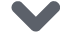# 硬货 | 手把手带你构建视频分类模型（附Python演练））

### 概述

• 了解如何使用计算机视觉和深度学习技术处理视频数据

• 我们将在Python中构建自己的视频分类模型

• 这是一个非常实用的视频分类教程，所以准备好Jupyter Notebook

• 视频分类概述

• 构建视频分类模型的步骤

• 探索视频分类数据集

• 训练视频分类模型

• 评估视频分类模型

### 构建视频分类模型的步骤

1. 浏览数据集并创建训练和验证集。我们将使用训练集来训练模型和验证集来评估模型

2. 从训练集以及验证集中的所有视频提取帧

3. 预处理这些帧，然后使用训练集中的帧来训练模型。使用验证集中的帧来评估模型

4. 一旦我们对验证集上的性能感到满意，就可以使用训练好的模型对新视频进行分类

### 探索视频分类数据集

unrar e UCF101.rar Videos/

UCF101的官方文件指出：

“在训练和测试中，将属于同一组的视频分开是非常重要的。由于组内的视频都是来自一个较长的视频，所以在训练集和测试集上共享来自同一组的视频可以获得较高的性能。”

import cv2   #捕获视频库import math  #数学操作库import matplotlib.pyplot as plt   #画图的库%matplotlib inlineimport pandas as pdfrom keras.preprocessing import image   # 预处理图像库import numpy as np    #数学操作库from keras.utils import np_utilsfrom skimage.transform import resize #改变图像尺寸from sklearn.model_selection import train_test_splitfrom glob import globfrom tqdm import tqdm

# 导入训练集txt文件，里面有视频名字列表f = open("trainlist01.txt", "r")temp = f.read()videos = temp.split('n')# 创建含有视频名字列表的dataframetrain = pd.DataFrame()train['video_name'] = videostrain = train[:-1]train.head()

# 导入测试集txt文件，里面有视频名字列表f = open("testlist01.txt", "r")temp = f.read()videos = temp.split('n')# 创建含有视频名字列表的dataframetest = pd.DataFrame()test['video_name'] = videostest = test[:-1]test.head()

# 为训练数据集创建标签train_video_tag = []for i in range(train.shape):    train_video_tag.append(train['video_name'][i].split('/'))train['tag'] = train_video_tag# 为测试数据集创建标签test_video_tag = []for i in range(test.shape):    test_video_tag.append(test['video_name'][i].split('/'))test['tag'] = test_video_tag

# 存储训练集视频的帧for i in tqdm(range(train.shape)):    count = 0    videoFile = train['video_name'][i]    cap = cv2.VideoCapture('UCF/'+videoFile.split(' ').split('/'))   # 从给定路径获取视频    frameRate = cap.get(5) #帧率    x=1    while(cap.isOpened()):        frameId = cap.get(1) #当前帧编号        ret, frame = cap.read()        if (ret != True):            break        if (frameId % math.floor(frameRate) == 0):            # 存储在train_1文件夹            filename ='train_1/' + videoFile.split('/').split(' ') +"_frame%d.jpg" % count;count+=1            cv2.imwrite(filename, frame)    cap.release()

# 获取所有图像的名字images = glob("train_1/*.jpg")train_image = []train_class = []for i in tqdm(range(len(images))):    # 创建图像名    train_image.append(images[i].split('/'))    # 创建图像类标    train_class.append(images[i].split('/').split('_'))# 存储在dataframe里train_data = pd.DataFrame()train_data['image'] = train_imagetrain_data['class'] = train_class# 转换dataframe为csv文件train_data.to_csv('UCF/train_new.csv',header=True, index=False)

### 训练视频分类模型

1. 读取我们之前为训练提取的所有帧

2. 创建一个验证集，它将帮助我们检查模型在看不见的数据上的表现

3. 定义模型的结构

4. 最后，训练模型并保存其权重

#### 读取所有视频帧

import kerasfrom keras.models import Sequentialfrom keras.applications.vgg16 import VGG16from keras.layers import Dense, InputLayer, Dropout, Flattenfrom keras.layers import Conv2D, MaxPooling2D, GlobalMaxPooling2Dfrom keras.preprocessing import imageimport numpy as npimport pandas as pdimport matplotlib.pyplot as pltfrom tqdm import tqdmfrom sklearn.model_selection import train_test_split

train = pd.read_csv('UCF/train_new.csv')train.head()

# 创建空列表train_image = []# 循环读取和保存帧for i in tqdm(range(train.shape)):    # 载入图片    img = image.load_img('train_1/'+train['image'][i], target_size=(224,224,3))    # 转换为array    img = image.img_to_array(img)    # 标准化像素值    img = img/255    # 保存到train_image列表    train_image.append(img)# 转换为numpy数组X = np.array(train_image)# 输出形状X.shape

#### 创建验证集

# 分离数据集y = train['class']# 创建训练与测试集X_train, X_test, y_train, y_test = train_test_split(X, y, random_state=42, test_size=0.2, stratify = y)

y_train = pd.get_dummies(y_train)y_test = pd.get_dummies(y_test)

#### 定义视频分类模型的结构

# 创建预训练的VGG16基本模型base_model = VGG16(weights='imagenet', include_top=False)

# 从训练集的帧中提取特征X_train = base_model.predict(X_train)X_train.shape

# 从验证集的帧中提取特征X_test = base_model.predict(X_test)X_test.shape

X_train = X_train.reshape(59075, 7*7*512)X_test = X_test.reshape(14769, 7*7*512)

# 标准化像素值max = X_train.max()X_train = X_train/maxX_test = X_test/max

# 图像形状X_train.shape

#定义结构model = Sequential()model.add(Dense(1024, activation='relu', input_shape=(25088,)))model.add(Dropout(0.5))model.add(Dense(512, activation='relu'))model.add(Dropout(0.5))model.add(Dense(256, activation='relu'))model.add(Dropout(0.5))model.add(Dense(128, activation='relu'))model.add(Dropout(0.5))model.add(Dense(101, activation='softmax'))

#### 训练视频分类模型

# 保存权重函数from keras.callbacks import ModelCheckpointmcp_save = ModelCheckpoint('weight.hdf5', save_best_only=True, monitor='val_loss', mode='min')

# 编译模型model.compile(loss='categorical_crossentropy',optimizer='Adam',metrics=['accuracy'])

# 训练模型model.fit(X_train, y_train, epochs=200, validation_data=(X_test, y_test), callbacks=[mcp_save], batch_size=128)

### 评估视频分类模型

1. 定义模型结构并加载权重

2. 创建测试数据

3. 对测试视频进行预测

4. 最后，评估模型

#### 定义模型结构并加载权重

from keras.models import Sequentialfrom keras.layers import Dense, Dropout, Flattenfrom keras.layers import Conv2D, MaxPooling2Dfrom keras.preprocessing import imageimport numpy as npimport pandas as pdfrom tqdm import tqdmfrom keras.applications.vgg16 import VGG16import cv2import mathimport osfrom glob import globfrom scipy import stats as s

base_model = VGG16(weights='imagenet', include_top=False)

model = Sequential()model.add(Dense(1024, activation='relu', input_shape=(25088,)))model.add(Dropout(0.5))model.add(Dense(512, activation='relu'))model.add(Dropout(0.5))model.add(Dense(256, activation='relu'))model.add(Dropout(0.5))model.add(Dense(128, activation='relu'))model.add(Dropout(0.5))model.add(Dense(101, activation='softmax'))

#载入权重model.load_weights("weights.hdf5")

# 编译模型model.compile(loss='categorical_crossentropy',optimizer='Adam',metrics=['accuracy'])

#### 创建测试数据

# 获取测试列表f = open("testlist01.txt", "r")temp = f.read()videos = temp.split('n')# 创建dataframetest = pd.DataFrame()test['video_name'] = videostest = test[:-1]test_videos = test['video_name']test.head()

# 创建标签train = pd.read_csv('UCF/train_new.csv')y = train['class']y = pd.get_dummies(y)

#### 测试视频的预测

1. 首先，我们将创建两个空列表,一个用于存储预测标签，另一个用于存储实际标签

2. 然后，我们将从测试集中获取每个视频，提取该视频的帧并将其存储在一个文件夹中(在当前目录中创建一个名为temp的文件夹来存储帧)。我们将在每次迭代时从此文件夹中删除所有其他文件

3. 接下来，我们将读取temp文件夹中的所有帧，使用预先训练的模型提取这些帧的特征，进行预测得到标签后将其附加到第一个列表中

4. 我们将在第二个列表中为每个视频添加实际标签

# 创建两个列表来存储预测的和实际的标签predict = []actual = []# for循环从每个测试视频中提取帧for i in tqdm(range(test_videos.shape)):    count = 0    videoFile = test_videos[i]    cap = cv2.VideoCapture('UCF/'+videoFile.split(' ').split('/'))   # 从给定路径获取视频    frameRate = cap.get(5) #帧率    x=1    # 从临时文件夹中删除所有其他文件    files = glob('temp/*')    for f in files:        os.remove(f)    while(cap.isOpened()):        frameId = cap.get(1) #当前帧编号        ret, frame = cap.read()        if (ret != True):            break        if (frameId % math.floor(frameRate) == 0):            # 将此特定视频的帧存储在temp文件夹中            filename ='temp/' + "_frame%d.jpg" % count;count+=1            cv2.imwrite(filename, frame)    cap.release()    # 从临时文件夹中读取所有帧    images = glob("temp/*.jpg")    prediction_images = []    for i in range(len(images)):        img = image.load_img(images[i], target_size=(224,224,3))        img = image.img_to_array(img)        img = img/255        prediction_images.append(img)    # 将测试视频的所有帧转换为numpy数组    prediction_images = np.array(prediction_images)    # extracting features using pre-trained model    prediction_images = base_model.predict(prediction_images)    # 使用预训练模型提取特征    prediction_images = prediction_images.reshape(prediction_images.shape, 7*7*512)    # 转换一维数组中的特征    prediction = model.predict_classes(prediction_images)    # 在预测列表中添加预测模式，将标签分配给视频    predict.append(y.columns.values[s.mode(prediction)])    # 添加上视频的真实标签    actual.append(videoFile.split('/').split('_'))

#### 评估模型

from sklearn.metrics import accuracy_scoreaccuracy_score(predict, actual)*100

### 结束点击下方 |  | 了解更多